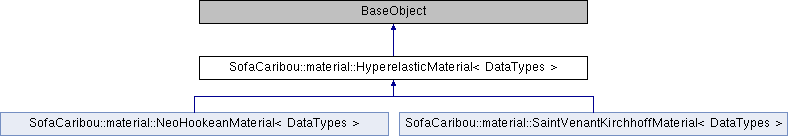Caribou
SofaCaribou::material::HyperelasticMaterial< DataTypes > Class Template Referenceabstract
Inheritance diagram for SofaCaribou::material::HyperelasticMaterial< DataTypes >:## Public Member Functions

SOFA_CLASS (SOFA_TEMPLATE(HyperelasticMaterial, DataTypes), sofa::core::objectmodel::BaseObject)

virtual void before_update ()
This is called just before the material is updated on every points (usually just before a Newton step). More...

virtual Real strain_energy_density (const Real &J, const Eigen::Matrix< Real, Dimension, Dimension > &C) const =0
Get the strain energy density Psi from the right Cauchy-Green strain tensor C.

virtual Eigen::Matrix< Real, Dimension, Dimension > PK2_stress (const Real &J, const Eigen::Matrix< Real, Dimension, Dimension > &C) const =0
Get the second Piola-Kirchhoff stress tensor from the right Cauchy-Green strain tensor C. More...

virtual Eigen::Matrix< Real, 6, 6 > PK2_stress_jacobian (const Real &J, const Eigen::Matrix< Real, Dimension, Dimension > &C) const =0
Get the jacobian of the second Piola-Kirchhoff stress tensor w.r.t the right Cauchy-Green strain tensor C. More...

## Static Public Member Functions

static std::string templateName (const HyperelasticMaterial< DataTypes > *=nullptr)
Return the data type (ex. More...

static bool canCreate (HyperelasticMaterial< DataTypes > *o, sofa::core::objectmodel::BaseContext *context, sofa::core::objectmodel::BaseObjectDescription *arg)
Check if we can create a HyperelasticMaterial<DataTypes> with the given template argument (from the scene parser). More...

## ◆ before_update()

template<class DataTypes >
 virtual void SofaCaribou::material::HyperelasticMaterial< DataTypes >::before_update ( )
inlinevirtual

This is called just before the material is updated on every points (usually just before a Newton step).

It can be used to update some coefficients that will be used on material points (for example, compute the parameters mu and lambda from the young modulus and poisson ratio given as data arguments).

## ◆ canCreate()

template<class DataTypes >
 static bool SofaCaribou::material::HyperelasticMaterial< DataTypes >::canCreate ( HyperelasticMaterial< DataTypes > * o, sofa::core::objectmodel::BaseContext * context, sofa::core::objectmodel::BaseObjectDescription * arg )
inlinestatic

Check if we can create a HyperelasticMaterial<DataTypes> with the given template argument (from the scene parser).

If no template argument was specified to the component, try to find a mechanical state in the current context to deduce the data type.

## ◆ PK2_stress()

template<class DataTypes >
 virtual Eigen::Matrix SofaCaribou::material::HyperelasticMaterial< DataTypes >::PK2_stress ( const Real & J, const Eigen::Matrix< Real, Dimension, Dimension > & C ) const
pure virtual

Get the second Piola-Kirchhoff stress tensor from the right Cauchy-Green strain tensor C.

With the energy density function Psi(C) -> scalar the second Piola-Kirchhoff stress tensor S is defined as S = 2*d(Psi)/dC and is a symmetric second order matrix (eg. 3x3 matrix).

## ◆ PK2_stress_jacobian()

template<class DataTypes >
 virtual Eigen::Matrix SofaCaribou::material::HyperelasticMaterial< DataTypes >::PK2_stress_jacobian ( const Real & J, const Eigen::Matrix< Real, Dimension, Dimension > & C ) const
pure virtual

Get the jacobian of the second Piola-Kirchhoff stress tensor w.r.t the right Cauchy-Green strain tensor C.

With the energy density function Psi(C) -> scalar the jacobian of the second Piola-Kirchhoff stress tensor S is defined as J = 2*d^2(Psi)/dC^2 = d(S)/dC and is a symmetric fourth order matrix represented in its compressed format (eg. 6x6 matrix).

## ◆ templateName()

template<class DataTypes >
 static std::string SofaCaribou::material::HyperelasticMaterial< DataTypes >::templateName ( const HyperelasticMaterial< DataTypes > * = `nullptr` )
inlinestatic

Return the data type (ex.

Vec3D) as the template name

The documentation for this class was generated from the following file: